Multiplying Fractions by Fractions Math Videos for Kids - Grades 3-5
1%
It was processed successfully!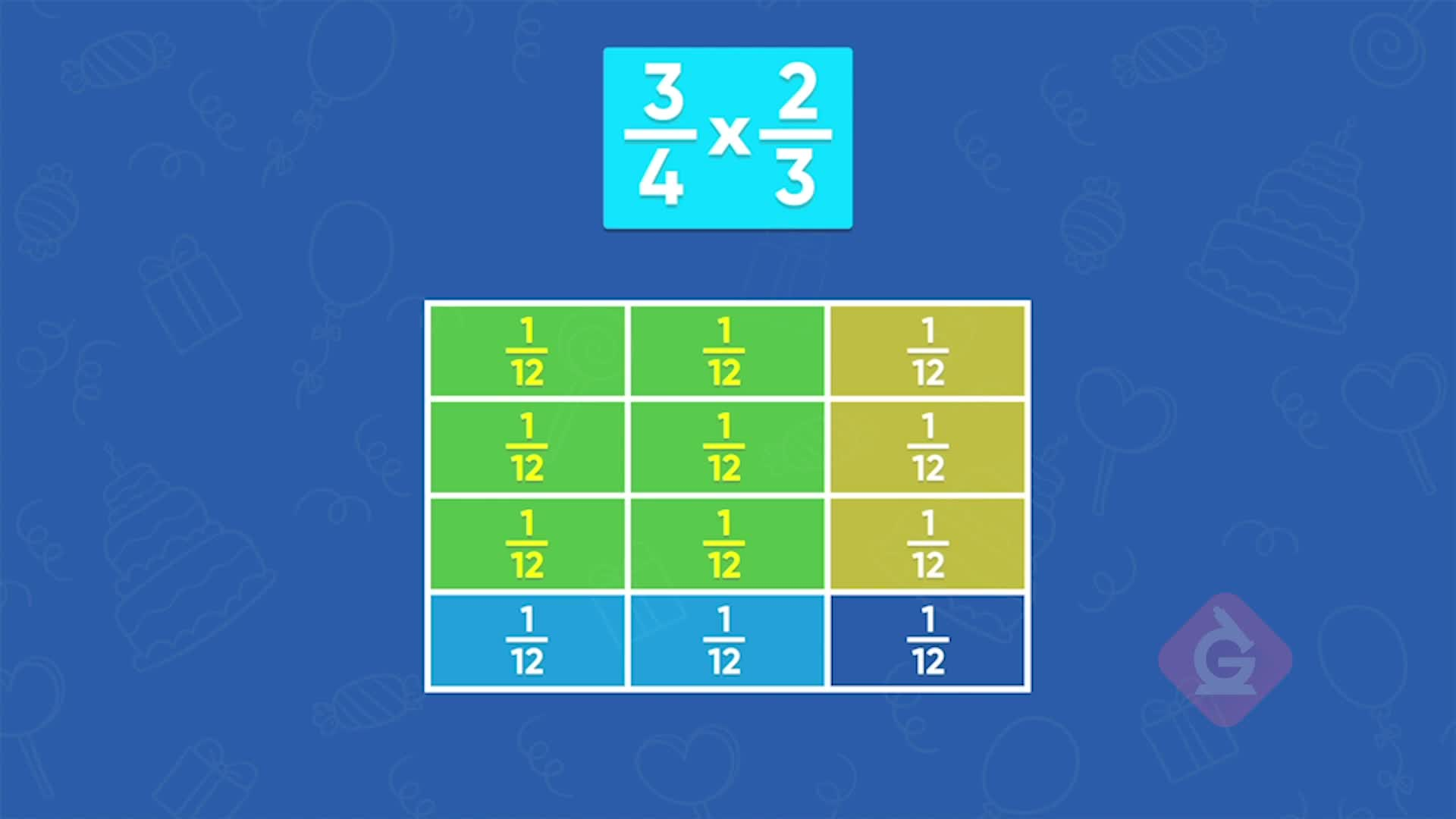WHAT IS MULTIPLYING FRACTIONS BY FRACTIONS?

Previously, you multiplied fractions by whole numbers and whole numbers by fractions. You can multiply fractions by fractions using a very similar process.

To better understand multiplying fractions by fractions…

WHAT IS MULTIPLYING FRACTIONS BY FRACTIONS?. Previously, you multiplied fractions by whole numbers and whole numbers by fractions. You can multiply fractions by fractions using a very similar process. To better understand multiplying fractions by fractions…

## LET’S BREAK IT DOWN!

### Birthday Cake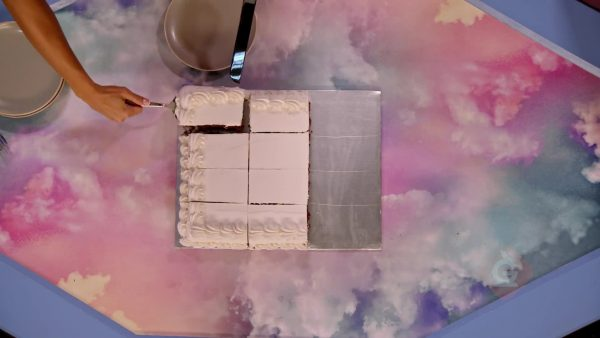Let’s say you have two-thirds of a birthday cake and you want to find out how much will be left if you eat [ggfrac]3/4[/ggfrac] of what is left. You can model this by dividing a rectangle into 3 equal parts with vertical lines. Then color in two of those parts with color 1. Now you can divide the rectangle into fourths using horizontal lines. You can use a different color to color in 3 of those 4 parts to represent [ggfrac]3/4[/ggfrac]. The part that remains colored by color 1 but not color 2 is the amount of cake that will be left after you eat [ggfrac]3/4[/ggfrac] of [ggfrac]2/3[/ggfrac] of a birthday cake. The remaining section is [ggfrac]2/12[/ggfrac] or [ggfrac]1/6[/ggfrac] of the whole cake. Try this one yourself. You have [ggfrac]4/5[/ggfrac] of a birthday cake left. You eat [ggfrac]1/3[/ggfrac] of what is left. How much of the whole cake is left now?

Birthday Cake Let’s say you have two-thirds of a birthday cake and you want to find out how much will be left if you eat [ggfrac]3/4[/ggfrac] of what is left. You can model this by dividing a rectangle into 3 equal parts with vertical lines. Then color in two of those parts with color 1. Now you can divide the rectangle into fourths using horizontal lines. You can use a different color to color in 3 of those 4 parts to represent [ggfrac]3/4[/ggfrac]. The part that remains colored by color 1 but not color 2 is the amount of cake that will be left after you eat [ggfrac]3/4[/ggfrac] of [ggfrac]2/3[/ggfrac] of a birthday cake. The remaining section is [ggfrac]2/12[/ggfrac] or [ggfrac]1/6[/ggfrac] of the whole cake. Try this one yourself. You have [ggfrac]4/5[/ggfrac] of a birthday cake left. You eat [ggfrac]1/3[/ggfrac] of what is left. How much of the whole cake is left now?

### Mac N Cheese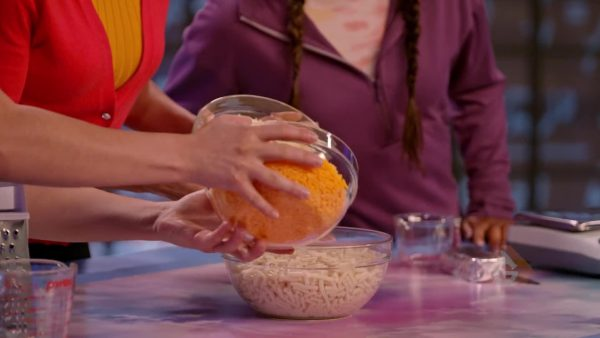Let’s say you have a recipe for macaroni and cheese that calls for [ggfrac]5/6[/ggfrac] of a pound of cheese. You know that [ggfrac]3/5[/ggfrac] of the cheese must be cheddar cheese. You want to find out how many pounds of cheddar cheese you need. Find [ggfrac]3/5[/ggfrac] of [ggfrac]5/6[/ggfrac] by multiplying [ggfrac]3/5[/ggfrac] times [ggfrac]5/6[/ggfrac]. Take a square and divide it vertically into 6 equal sections. Shade 5 out of the 6 sections blue. Now split the square horizontally into 5 equal sections and highlight 3 of the sections yellow. The overlapping sections in green represent the product. Each part of the square is [ggfrac]1/30[/ggfrac] of the square and 15 of those parts are shaded green. You need [ggfrac]15/30[/ggfrac] or [ggfrac]1/2[/ggfrac] pound of cheddar cheese. You can also get that answer by multiplying the denominators. [ggfrac]5/6[/ggfrac] x [ggfrac]3/5[/ggfrac] = [ggfrac]15/30[/ggfrac] = [ggfrac]1/2[/ggfrac]. Try this one yourself. Another recipe calls for [ggfrac]1/2[/ggfrac] pound of cheese and [ggfrac]3/8[/ggfrac] of that must be cheddar cheese. How much cheddar cheese do you need?

Mac N Cheese Let’s say you have a recipe for macaroni and cheese that calls for [ggfrac]5/6[/ggfrac] of a pound of cheese. You know that [ggfrac]3/5[/ggfrac] of the cheese must be cheddar cheese. You want to find out how many pounds of cheddar cheese you need. Find [ggfrac]3/5[/ggfrac] of [ggfrac]5/6[/ggfrac] by multiplying [ggfrac]3/5[/ggfrac] times [ggfrac]5/6[/ggfrac]. Take a square and divide it vertically into 6 equal sections. Shade 5 out of the 6 sections blue. Now split the square horizontally into 5 equal sections and highlight 3 of the sections yellow. The overlapping sections in green represent the product. Each part of the square is [ggfrac]1/30[/ggfrac] of the square and 15 of those parts are shaded green. You need [ggfrac]15/30[/ggfrac] or [ggfrac]1/2[/ggfrac] pound of cheddar cheese. You can also get that answer by multiplying the denominators. [ggfrac]5/6[/ggfrac] x [ggfrac]3/5[/ggfrac] = [ggfrac]15/30[/ggfrac] = [ggfrac]1/2[/ggfrac]. Try this one yourself. Another recipe calls for [ggfrac]1/2[/ggfrac] pound of cheese and [ggfrac]3/8[/ggfrac] of that must be cheddar cheese. How much cheddar cheese do you need?

### Puppets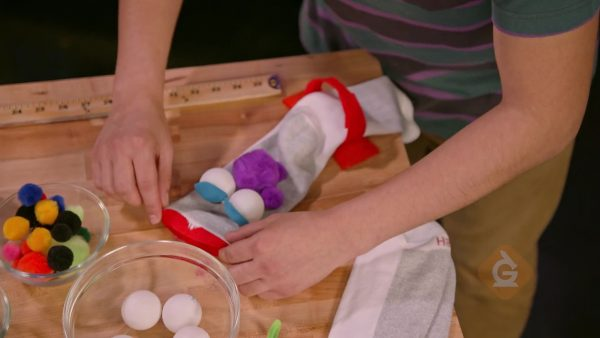Let’s say the instructions to make a puppet say you need a piece of felt that is [ggfrac]1/4[/ggfrac] yard long. However, your teacher asks you to use a piece that is 2[ggfrac]2/3[/ggfrac] times longer, to make a bigger puppet. To find the length of felt that you need, find the product of 2[ggfrac]2/3[/ggfrac] x [ggfrac]1/4[/ggfrac]. First, convert 2[ggfrac]2/3[/ggfrac] into a fraction greater than 1: [ggfrac]8/3[/ggfrac]. Next, find [ggfrac]8/3[/ggfrac] x [ggfrac]1/4[/ggfrac] by multiplying the numerators and then the denominators:[ggfrac]8/3[/ggfrac] x [ggfrac]1/4[/ggfrac] = [ggfrac]8x1/3x4[/ggfrac] = [ggfrac]8/12[/ggfrac]. The piece of felt should be [ggfrac]8/12[/ggfrac] yard long. Try this one yourself. You need a piece of felt that is 1[ggfrac]3/4[/ggfrac] times longer than [ggfrac]1/8[/ggfrac] yard. What is the length of the piece of felt you need?

Puppets Let’s say the instructions to make a puppet say you need a piece of felt that is [ggfrac]1/4[/ggfrac] yard long. However, your teacher asks you to use a piece that is 2[ggfrac]2/3[/ggfrac] times longer, to make a bigger puppet. To find the length of felt that you need, find the product of 2[ggfrac]2/3[/ggfrac] x [ggfrac]1/4[/ggfrac]. First, convert 2[ggfrac]2/3[/ggfrac] into a fraction greater than 1: [ggfrac]8/3[/ggfrac]. Next, find [ggfrac]8/3[/ggfrac] x [ggfrac]1/4[/ggfrac] by multiplying the numerators and then the denominators:[ggfrac]8/3[/ggfrac] x [ggfrac]1/4[/ggfrac] = [ggfrac]8x1/3x4[/ggfrac] = [ggfrac]8/12[/ggfrac]. The piece of felt should be [ggfrac]8/12[/ggfrac] yard long. Try this one yourself. You need a piece of felt that is 1[ggfrac]3/4[/ggfrac] times longer than [ggfrac]1/8[/ggfrac] yard. What is the length of the piece of felt you need?

### Track Team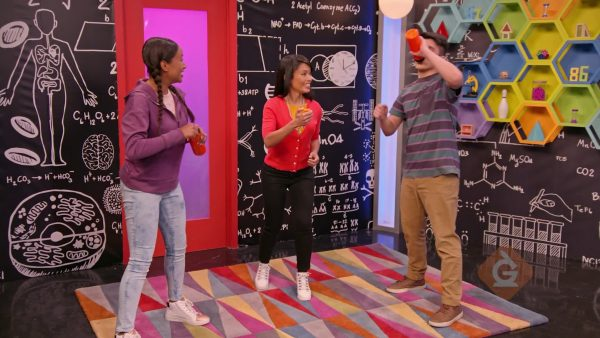Let's say you run 1[ggfrac]1/3[/ggfrac] miles every day. Your coach tells you that you need to run 2[ggfrac]1/4[/ggfrac] times that distance. You want to find out how far you need to run each day now. You need to find 1[ggfrac]1/3[/ggfrac] x 2[ggfrac]1/4[/ggfrac]. First, convert both mixed numbers to fractions greater than 1: 1[ggfrac]1/3[/ggfrac] = [ggfrac]4/3[/ggfrac] and 2[ggfrac]1/4[/ggfrac] = [ggfrac]9/4[/ggfrac]. Now you can find the product: [ggfrac]4/3[/ggfrac] x [ggfrac]9/4[/ggfrac] = [ggfrac]36/12[/ggfrac] = 3. You need to run 3 miles each day. Try this one yourself. Your friend runs 1[ggfrac]1/2[/ggfrac] miles each day but his coach tells him he needs to run 1[ggfrac]3/4[/ggfrac] times that distance. What distance does your friend need to run?

Track Team Let's say you run 1[ggfrac]1/3[/ggfrac] miles every day. Your coach tells you that you need to run 2[ggfrac]1/4[/ggfrac] times that distance. You want to find out how far you need to run each day now. You need to find 1[ggfrac]1/3[/ggfrac] x 2[ggfrac]1/4[/ggfrac]. First, convert both mixed numbers to fractions greater than 1: 1[ggfrac]1/3[/ggfrac] = [ggfrac]4/3[/ggfrac] and 2[ggfrac]1/4[/ggfrac] = [ggfrac]9/4[/ggfrac]. Now you can find the product: [ggfrac]4/3[/ggfrac] x [ggfrac]9/4[/ggfrac] = [ggfrac]36/12[/ggfrac] = 3. You need to run 3 miles each day. Try this one yourself. Your friend runs 1[ggfrac]1/2[/ggfrac] miles each day but his coach tells him he needs to run 1[ggfrac]3/4[/ggfrac] times that distance. What distance does your friend need to run?

## MULTIPLYING FRACTIONS BY FRACTIONS VOCABULARY

Fraction
Represents a part of a whole.
Mixed number
A number made up of a whole number and a fraction.
Numerator
The number above the line in a fraction, which tells how many equal parts of the whole the fraction represents.
Denominator
The number below the line in a fraction, which tells how many equal parts are in the whole.
Area model
A shape (often a square) used to make a model of the whole and the operation used; in this case, multiplication of a fraction by a fraction.
Convert
Change the form of a mixed number to a fraction greater than 1 or a fraction greater than one to a mixed number.

## MULTIPLYING FRACTIONS BY FRACTIONS DISCUSSION QUESTIONS

### How can you model [ggfrac]2/5[/ggfrac] x [ggfrac]3/4[/ggfrac]? Find the product.

Divide a square into fifths horizontally. Shade two of the fifths blue. Divide the square into fourths vertically. Shade three of the fourths yellow. The part of the square that is green (both blue and yellow) is the answer. [ggfrac]2/5[/ggfrac] x [ggfrac]3/4[/ggfrac] = [ggfrac]6/20[/ggfrac]

### Safa says that the product [ggfrac]2/3[/ggfrac] x [ggfrac]1/3[/ggfrac] is [ggfrac]3/3[/ggfrac]. Is she correct? Why or why not?

No. I think Safa used the rule for the addition of fractions rather than the rule for the multiplication of fractions. The correct product is [ggfrac]2/9[/ggfrac].

### What rule do you use to multiply a fraction by a fraction? Give an example.

Multiply the numerators to get the numerator of the answer. Multiply the denominators to get the denominator of the answer. Example: [ggfrac]3/7[/ggfrac] x [ggfrac]1/2[/ggfrac] = [ggfrac]3/14[/ggfrac]

### How do you multiply a mixed number by a mixed number? Give an example.

Convert both mixed numbers to fractions greater than 1. Then multiply using the rule for multiplying two fractions: multiply the numerators to get the numerator of the answer, and multiply the denominators to get the denominator of the answer. Example: 1[ggfrac]1/2[/ggfrac] x 3[ggfrac]2/3[/ggfrac] = [ggfrac]3/2[/ggfrac] x [ggfrac]11/3[/ggfrac] = [ggfrac]33/6[/ggfrac] = 5[ggfrac]3/6[/ggfrac] = 5[ggfrac]1/2[/ggfrac]

### How is multiplying a fraction by a fraction the same as or different from multiplying a fraction by a whole number?

To multiply a fraction by a fraction we need to multiply the numerators and multiply the denominators. When one number is a whole number, we do not need to multiply the denominators. This is because a whole number has a denominator of 1.
X

## Success

We’ve sent you an email with instructions how to reset your password.
Ok

## Choose Your Free Trial Period### 7 DaysContinue to Lessons### 90 DaysGet 90 days free by inviting other teachers to try it too.

Share with Teachers

## Get 90 Days Free

### By inviting 4 other teachers to try it too.

4 required

Skip, I will use a 7 days free trial

## Thank You!

Enjoy your free 90 days trial